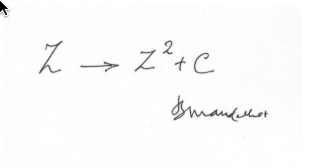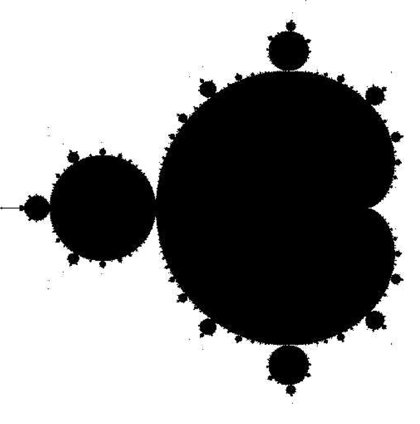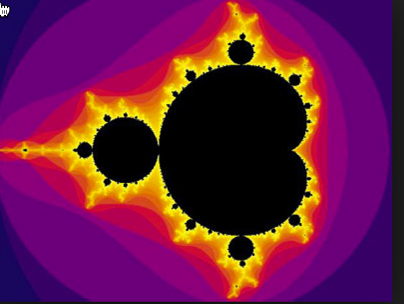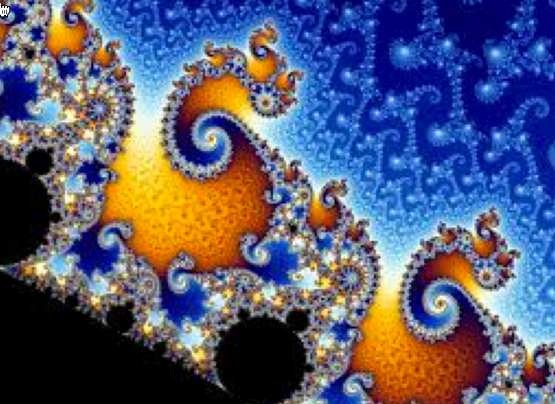Generating Mandelbrot Images¶

The mathematician Benoit Mandelbrot, studied the properties of complex numbers and discovered an interesting phenomena about them. His discovery led to the creation of Mandelbrot images, so named by Adrien Douady in honor of Mandelbrot’s work. These images are easy to generate, and result in very cool graphics images. Furthermore, the calculations needed to produce these images are an ideal candidate for exploring modern graphics hardware and parallel processing techniques. In short, they are worth a bit of study by any computer science student!

Complex Numbers¶

As we have seen, a complex number is one that has a “real” part and an “imaginary” part. The real part is just some floating point number. The imaginary part is another floating point number multiplied by the square root of -1. Now, every math student knows you cannot take the square root of -1, but if you could we would name it “i” and call it imaginary! We multiply “i” by some floating point number, add it to a real number and we get a complex number!Plotting Complex Numbers¶

Complex numbers have some pretty cool applications in engineering, science and math. We will not go into that here. What we will do it plot these numbers on a graph.

If we use the real part of a complex number to specify some “X” value on a graph, and the imaginary part of a complex number to point to some “Y” value on that graph, we can place any complex number somewhere on a conventional X-Y graph. The origin of this X-Y coordinate system corresponds to the complex number 0 + 0i.

The length of a line from the origin of the graph to the point is called the magnitude of the complex number. It is calculated using this formula:Complex Number Math¶

We can use the normal rules of math to figure out the basic math operations on complex numbers. All we need to remember is that!

When we work with these numbers, we do normal math, except when we multiply things together. If we ever generate, we replace that with -1.

Adding complex numbers is simple:Multiplying two complex numbers gives us this:Mandelbrot Sets¶

Mandelbrot’s discovery was pretty amazing. All he did was to take any point on the complex plain and use that number to do a simple calculation using something called a mathematical series.

Basically, you start with one number, plug it into the formula for the series to generate a new complex number. You plug this new number back into the same formula and generate a third complex number. Keep repeating this process as long as you like (computers are great at this!).Mandelbrot calculated the magnitude of the complex numbers and discovered that some headed off to infinity, and some did not. Those that headed off to infinity he tossed out, those that stayed bounded he included in a mathematical “set” of numbers. To keep this simple, he used integer numbers for his calculations.

When he plotted all of the complex numbers he ran through is formula, he colored the ones that stayed “bounded” black, and every number that headed out to infinity black.

This is what he saw:Wild, huh! His discovery started a bunch of folks off studying what was going on, and how to visualize it better.

Cooler Images¶

Eventually folks came up with a way to generate even cooler images. They counted the number of iterations (passes through the formula) and watched the magnitude of the numbers the series generated. If the magnitude keep growing larger, they recorded the count when the magnitude exceeded some magnitude like 2.0. They used this number in a variety of creative ways to generate a color. If the complex number was part of Mandelbrot’s set, they colored that spot black.

Then they started seeing images that looked like this:This finding started a revolution in computer graphics studies. Playing with the color scheme, and zooming around on this image, magnifying it as you go can result in some amazing images. Like this, for example:Parallel Processing¶

Generating a color for every pixel on a computer screen involves working through Mandelbrot’s series in a loop and watching what happens. The math involved in this process is completely self contained. It depends on nothing but that initial value for the complex number represented by a single point on the graph. That means if we have a ton of processors around, each could be processing a single pixel color at the same time. Well, except for computers like the Yosemite Supercomputer in Cheyenne, Wyoming, which has 77,000 processors, most of us only have a few cores in our computers, but we have many processing elements on our graphics cards. If we could figure out how to tap into that system, we could generate these images pretty fast.

That is exactly what computer science students have been doing for years now, as a way to explore the GPU in your system.

Perhaps we could explore that in our class, or maybe in a future class!